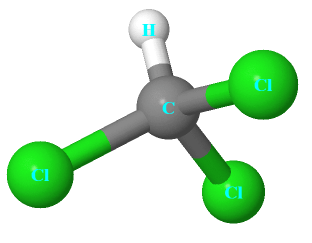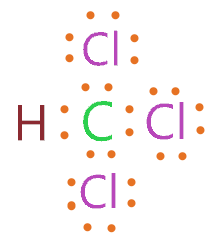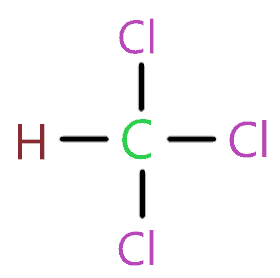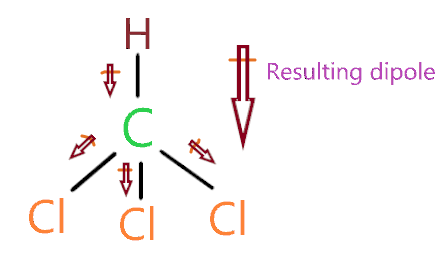# Chloroform (CHCl3) lewis dot structure, molecular geometry, polarity, hybridization

Home  > Chemistry Article > CHCl3 lewis structure and its molecular geometryChloroform is a colorless liquid made up of carbon, hydrogen, and chlorine with the chemical formula CHCl3. It is used as a solvent in the production of refrigerant freon. It is a highly volatile and dense liquid that feels cool to touch.

In this article, we will discuss CHCl3 lewis structure, molecular geometry, polar or non-polar, bond angle, etc.

It is one of the interesting molecules used for making someone unconscious or used as a sedative on the patient before surgery. A high concentration of this molecule can cause central nervous system dysfunction, nausea, or uneven liver function.

Properties of Chloroform

• It is a colorless liquid with an ethereal odor.
• It is denser than water and soluble in water.
• Under most conditions, it is non-flammable.
• It has a molar mass of 119.37 g·mol−1.
• It has a boiling point of 61.15 °C and a melting point of −63.5 °C.
 Name of Molecule Chloroform or Trichloromethane Chemical formula CHCl3 Molecular geometry of CHCl3 Tetrahedral Electron geometry of CHCl3 Tetrahedral Hybridization Sp³ Bond angle 109.5º Total Valence electron in CHCl3 lewis structure 26 Formal charge 0
Page Contents

## How to draw CHCl3 lewis structure?

CHCl3 lewis structure contains one hydrogen, one carbon, and three chlorine atom. No lone pair is present on the central atom of the CHCl3 lewis structure. Each chlorine contains 3 lone pairs and is spaced evenly around the central atom.

Let’s see how to draw CHCl3 lewis dot structure step by step-

## Follow some steps for drawing the lewis dot structure of CHCl3

1. Count total valence electron in CHCl3

In the first step, we will calculate how many valence electrons are present for CHCl3 so that we can arrange them in the lewis diagram. For this look at the periodic group of each atom.

As carbon belongs to the 14th periodic group, chlorine to the 17th, and hydrogen to the first group. Therefore the valence electron for carbon is 4, for chlorine 7, and for hydrogen, it is 1.

⇒ Total valence electron in Carbon = 4

⇒ Total valence electron in Chlorine = 7

⇒ Total valence electron in Hydrogen = 1

∴ Total valence electron available for drawing the CHCl3 lewis structure = 4 + 1 +  7*3 = 26 electrons     [∴CHCl3 has three chlorine atom]2. Find the least electronegative atom and placed it at center

Now we need to find the least electronegative atom in the CHCl3 molecule for placing at it the center of the lewis diagram. Remember hydrogen always go outside in structure. Among carbon and chlorine, carbon is less electronegative because electronegativity increase from left to right in the periodic table.

Therefore place carbon at the center in the lewis diagram and chlorine or hydrogen spaced evenly around it.3. Connect outer atom to central atom with a single bond

In this step, we simply start drawing the CHCl3 skeletal structure by connecting the all outer atom(chlorine and hydrogen) to the central atom(carbon) with a single bond.Now we will find how many valence electrons we used till now. As one single bond contains two electrons and we used 4 single bonds in the above structure. So, a total of 8 electrons we used till now from 26 available valence electrons for CHCl3 lewis structure.

∴ (26 – 8) = 18 valence electrons

Therefore, we are left with 18 valence electrons more.

4. Placed remaining valence electrons starting from outer atom first

In this step, place these remaining 18 valence electrons around the outer atom first. In the CHCl3 molecule, chlorine and hydrogen are the outer atoms.

But hydrogen can only hold a maximum of two electrons in its valence shell. So, placed these remaining valence electrons around the chlorine atom to complete their octet shell.### CHCl3 lewis structure

As hydrogen needs only two electrons to complete its octet which is fulfilled by one single bond present between hydrogen and carbon. Chlorine needs 8 electrons in its valence shell for completing the octet.

By looking at the above structure, we see each chlorine atom has 8 electrons around them. So, chlorine and hydrogen fulfill their octet comfortably.

Also, the central atom(Carbon) has also completed its octet with the help of 4 single bonds. As each bond contains 2 electrons and carbon needs only 8 electrons to complete its octet.

Hence, we used all valence electrons available for drawing the CHCl3 lewis dot structure and completed the octet of all atoms comfortably.

## What are the electron and molecular geometry of CHCl3?

The molecular geometry of CHCl3 is tetrahedral and electron geometry is also tetrahedral as carbon being a central atom attached with four atoms and it has Sp3 hybridization.

Each chlorine has three lone pair that creates repulsion and tries to repel the bonded pair of electrons as a result electrons move away from each other that stretch the structure hence it shape become tetrahedral.

Let’s see how to find the molecular geometry of CHCl3 step by step-

## Follow some steps to find CHCl3 molecular geometry

1. Find the Number of lone pairs present on the central atom of the CHCl3 lewis structure

As we know overall shape of any molecule can be affected by the presence of lone pair on its central atom. According to the CHCl3 lewis structure, carbon is the central atom that has no lone pair on it.

Or you can check the lone pair on the central atom by the formula given below-So, carbon is the central atom that is attached to four atoms and has 4 valence electrons in its shell.

So, L.P. on carbon = (4 – 4)/2

= 0

Hence lone pair on the central atom of the CHCl3 lewis dot structure is 0.

### 2. Find hybridization number of CHCl3

Hybridization numbers can help to determine the hybridization of any atom or molecule. Generally, it is also called a steric number.

Use the given formula to find the hybridization number for CHCl3.

Hybridization number of CHCl3 = (Number of atoms attached to Carbon + Lone pair on Carbon)

In the CHCl3 molecule, carbon is the central atom that has no lone pair on it and is attached to 4 atoms(1 hydrogen + 3 chlorine).

So, H = 4 + 0

= 4

So, the hybridization number for CHCl3 we got 4 which means it has Sp³ hybridization.

### 3. Use VSEPR theory or AXN method to determine CHCl3 molecular geometry

According to Valence shell electron pair repulsion theory if the molecule contains Sp³ hybridization and 0 lone pair of electrons, then the shape of the molecule is tetrahedral.### CHCl3 molecular geometry

Now we will apply the AXN method to determine the shape of CHCl3 with the help of the VSEPR chart.As per CHCl3 lewis structure, AXN notation of it follow as-

• A = Carbon is a central atom.
• X = Four number of bonds(1 hydrogen + 3 Chlorine) attached to carbon.
• N = No lone pair present on the carbon atom.

So, the generic formula for CHCl3 is AX4.

According to the VSEPR chart, if a molecule central atom has 4 bonded atoms and no lone pair on it then the geometry of that molecule will be tetrahedral.

Therefore, CHCl3 molecular geometry is tetrahedral as it has 4 bonded atoms and no lone pair on it with Sp³ hybridization. And the generic formula for this is AX4.## Chloroform polarity: is CHCl3 polar or nonpolar

Is CHCl3 polar or non-polar? Yes, CHCl3 is a polar molecule with a net dipole moment of 1.15 D. It has two types of bonds such as C-H and three C-Cl and both of them generate dipole moment due to electronegativity difference between them.

As the molecule is not symmetric so dipole moments along these bonds cannot cancel each other making this molecule polar in nature.

CHCl3 is not a strongly polar molecule as other organic compounds because it has three symmetrical C-Cl bond that partially cancels out each other due to the tetrahedral geometry of CHCl3.

In chemistry, it is said molecule that has tetrahedral geometry are non-polar in nature but this condition valid only when all atoms in the molecule is same like CCl4 has four chlorine atom and that make four similar C-Cl bonds which cause non-polar nature of it.

But in the case of CHCl3, two different atoms present(hydrogen and chlorine) make different bonds and create some dipole moment which makes this molecule polar in nature.

## Three factors that indicate the polarity of CHCl3

1. Electronegativity:

If A atom is more electronegative than B, then A has more power to attract electrons towards itself. So, electronegativity defines an atom’s tendency to attract electrons towards itself. In CHCl3, electronegativity for carbon is 2.5, for hydrogen 2.1, and for chlorine, it is 3.16.

So, the electronegativity difference among the C-Cl bond is 0.66 and for the C-H bond, it is 0.4.

As per the Pauling scale, if the electronegativity between atoms is more than o.4, then the bond between those atoms is polar in nature.

2. Dipole moment

The net dipole moment of CHCl3 is 1.15 D which is not great but enough to make this molecule polar in nature. Dipole moment measures the strength of polarity between carbon, hydrogen, and chlorine atom.

As greater the dipole moment of the molecule, the more is the polar nature of that molecule.

In CHCl3, chlorine is more electronegative so it attracts electrons towards itself that induced some negative charge around it and some partial positive charge induced around the carbon atom. The direction of dipole moment generated along the C-Cl bond is from positive to negative charge.

Also, carbon is more electronegative than hydrogen so it attracts electrons towards itself from hydrogen sides.

Hence all these electron densities pulled away in direction of chlorine or near the chlorine that makes this molecule polar in nature because all dipole moments of bonds in the same direction will be added.3. Geometrical or molecular shape

Geometry structure can influence the polarity of the molecule because asymmetrical shape distributes unequal charge in the molecule so it becomes difficult to cancel out the dipole moment in the molecule.

As CHCl3 molecular geometry is tetrahedral and no lone pair is present on the central atom of it, this makes its geometry symmetrical.

But the only way a tetrahedral geometry can be asymmetrical is if one atom or more atoms of the molecule are different from the rest. So, in case of CHCl3, hydrogen and chlorine is the different atom.

Therefore, the molecular geometry of CHCl3 is asymmetrical with a tetrahedral shape and that makes this molecule polar in nature.

## What is the formal charge in CHCl3 lewis dot structure and how to calculate it?

The formal charge of CHCl3 shows that which atom(Carbon, hydrogen, or Chlorine) has more positive or negative charge present on it. When the formal charge is closest to zero of the molecule, then the lewis diagram of that molecule is most stable.

To calculate the formal charge in Chloroform lewis structure. Use this equation given below:

Formal charge = (Valence electrons – unbonded electrons – 1/2 bonded electrons)

Now we will calculate the formal on the central atom of the CHCl3 molecule which is carbon.

⇒ Valence electron of carbon = 4

⇒ Non-bonding electrons on carbon = 0

⇒ Bonding electrons attached to carbon = 8

∴ (4 – 0 – 8/2)

= 0 is the formal charge on carbon in CHCl3.

## FAQ

### How many total lone pairs are present in the CHCl3 lewis dot structure?

In the CHCl3 molecule, there is no lone pair present on the central atom. But each chlorine atom in CHCl3 has three lone pairs around it.

So, each chlorine has three lone pairs that mean a total of 9 lone pairs present in the CHCl3 lewis structure.

### Why molecular geometry of CHCl3 is same as its electron geometry?

The electron geometry of CHCl3 is tetrahedral and the molecular of it is also tetrahedral because electron geometry considers lone pair and bond pair of atoms to find the shape of the molecule but molecular geometry only considers bonded pairs of electrons to determine the shape of the molecule.

Also, there is no lone pair present on the central atom in the CHCl3 molecule. So, here electron geometry only considers bond pairs to determine the shape of CHCl3.

Hence the molecular geometry of CHCl3 is the same as its electron geometry.

## Summary

• The total valence electron available for drawing the chloroform (CHCl3) lewis structure is 26.
• The hybridization of chloroform is Sp³.
• The bond angle of CHCl3 is 109.5º.
• Chloroform is slightly polar in nature.
• The molecular geometry of CHCl3 is tetrahedral and its electron geometry is also tetrahedral.
• The net dipole moment of chloroform is 1.15 D.
• The total lone pair in the CHCl3 lewis dot structure is 9 but no lone pair is present on the central atom of it.
##### Subscribe to Blog via Email

Join 2 other subscribers

Share it...